Pinwheel Color Melt
Last updated: October 17, 2017

# Pinwheel Color Melt

ALL PHOTOS SLIDESHOW
GET THE HOW-TO• Formula A

Matrix Light Master + 20-volume developer

• Formula B

Matrix Color Sync 8M + 10-volume developer

• Formula C

Color Sync 10M + 10-volume developer# Pinwheel Color Melt

Color melting is today’s hottest trend, but don’t think it can only be done with fashion colors. This technique adds tons of dimension because of the pinwheel sectioning technique and uses blonde mocha shades for richness.

As a hairdresser at JCPenney Salon and The Salon by InStyle, you’ll learn versatile and useable techniques like this one to keep ahead of trends (and the education you receive is PAID). Plus, the company offers a storewide discount at JCPenney, medical, dental, paid time off and a 401k! And you can get up to \$1,000 in bonuses based on performance—so basically, you get paid extra for doing what you love. Learn more about the benefits of working at JCPenney Salon at jobs.jcp.com.

Creative Direction: Dilek Onur-Taylor, @dilekonurtaylor
Manufacturer: JCPenney Salon; Matrix, @matrix

## COLOR FORMULAS• Formula A

Matrix Light Master + 20-volume developer

• Formula B

Matrix Color Sync 8M + 10-volume developer

• Formula C

Color Sync 10M + 10-volume developer

## HOW-TO STEPS•• Formula A

Matrix Light Master + 20-volume developer

• Formula B

Matrix Color Sync 8M + 10-volume developer

• Formula C

Color Sync 10M + 10-volume developer

1

Create pinwheel sections off the crown by sectioning the head into quadrants and then dividing each quadrant in half. The front section should extend from the outside corner of each brow to the crown for a total of nine sections.

•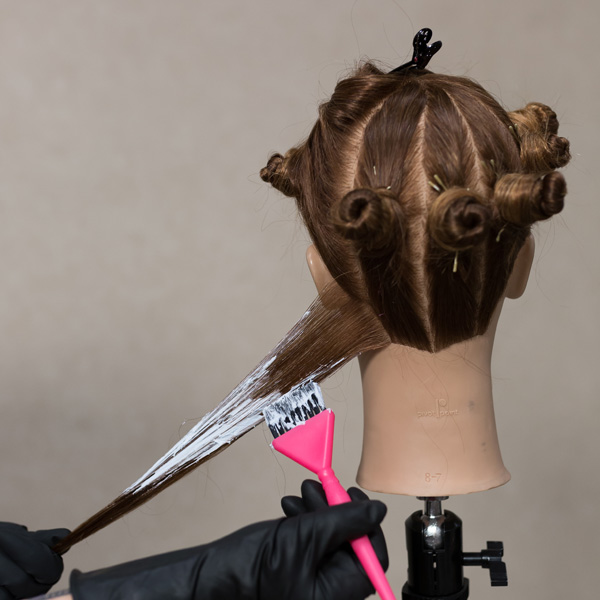• Formula A

Matrix Light Master + 20-volume developer

• Formula B

Matrix Color Sync 8M + 10-volume developer

• Formula C

Color Sync 10M + 10-volume developer

2

Release the back section. Create a diagonal zigzag subsection and hold it taut by pinching the ends. Load the brush with Formula A and place it in the center of the section, then feather the formula upward to diffuse the application.

•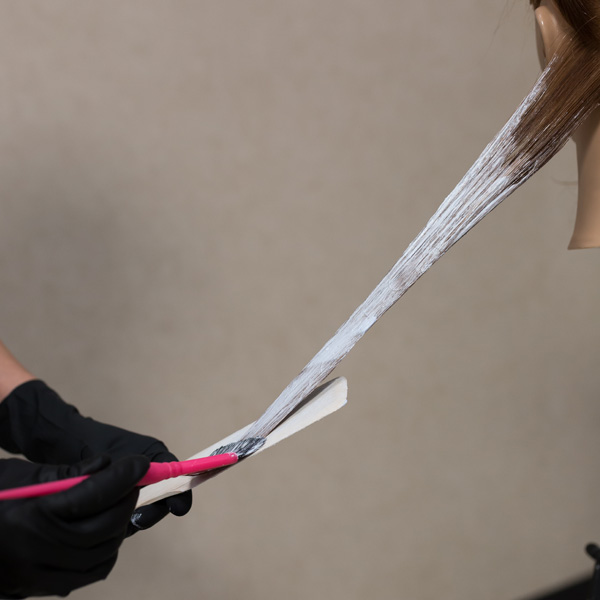• Formula A

Matrix Light Master + 20-volume developer

• Formula B

Matrix Color Sync 8M + 10-volume developer

• Formula C

Color Sync 10M + 10-volume developer

3

Place the ends on a balayage board and saturate with Formula A.

•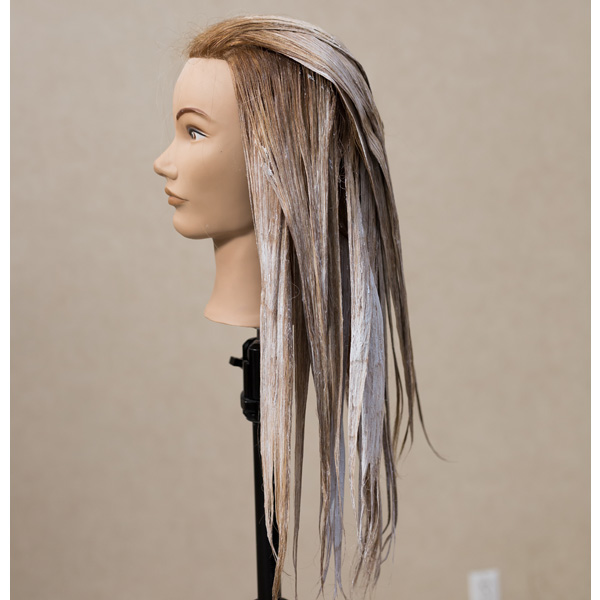• Formula A

Matrix Light Master + 20-volume developer

• Formula B

Matrix Color Sync 8M + 10-volume developer

• Formula C

Color Sync 10M + 10-volume developer

4

Work up the section until it’s complete, then continue working around the head in this manner. On the top section, create zigzag horizontal sections and overdirect the hair away from the face. Backcomb the front hairline section slightly before finishing the application of Formula A. Process, shampoo and dry the hair.

•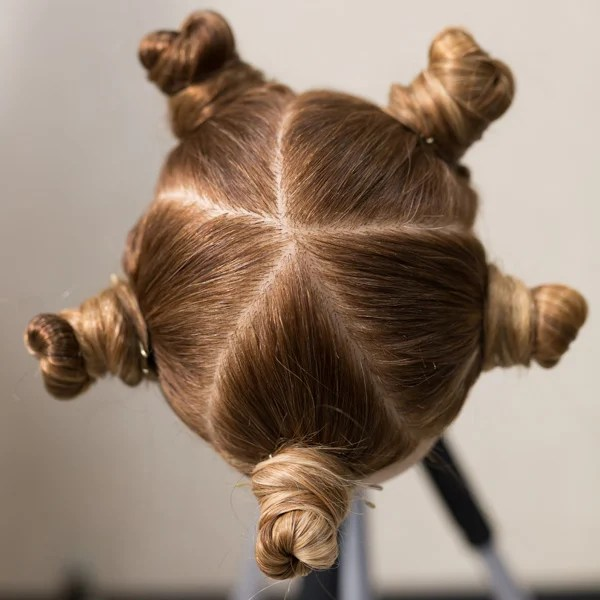• Formula A

Matrix Light Master + 20-volume developer

• Formula B

Matrix Color Sync 8M + 10-volume developer

• Formula C

Color Sync 10M + 10-volume developer

5

Resection the hair into five sections—a fringe section that extends from the corners of the eyes to a point on top, two side sections and two back sections.

•• Formula A

Matrix Light Master + 20-volume developer

• Formula B

Matrix Color Sync 8M + 10-volume developer

• Formula C

Color Sync 10M + 10-volume developer

6

Release the first section. Create a horizontal subsection, then apply Formula B from the base to the midshaft, top and bottom. Leaving a disconnected area between, apply Formula C from midshaft to ends. Connect the two formulas on the strand by placing a section between your index and middle fingers, then pressing and blending.

•• Formula A

Matrix Light Master + 20-volume developer

• Formula B

Matrix Color Sync 8M + 10-volume developer

• Formula C

Color Sync 10M + 10-volume developer

7

Complete the section in this manner, then complete the rest of the head. Process, shampoo, condition and style the hair.

•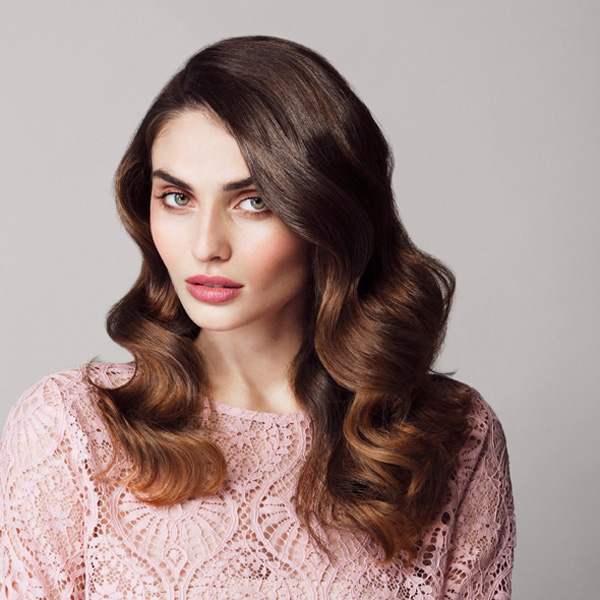• Formula A

Matrix Light Master + 20-volume developer

• Formula B

Matrix Color Sync 8M + 10-volume developer

• Formula C

Color Sync 10M + 10-volume developer

8

The finished look!